FIR & IIR

tulisan ini dibuat dalam usaha bertobat dalam menuju kuis PSD:

Finite impulse response

A finite impulse response (FIR) filter is a type of a digital filter. It is ‘finite’ because its response to an impulse ultimately settles to zero. This is in contrast to infinite impulse response filters which have internal feedback and may continue to respond indefinitely.

We start the discussion by stating the difference equation which defines how the input signal is related to the output signal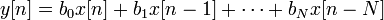$y[n]=b_0 x[n] + b_1 x[n-1] + cdots + b_N x[n-N]$

where x[n] is the input signal, y[n] is the output signal and bi are the filter coefficients. N is known as the filter order; an Nth-order filter has (N + 1) terms on the right-hand side; these are commonly referred to as taps.

The previous equation can also be expressed as$y[n] = sum_{i=0}^{N} b_i x[n-i].$

To find the impulse response we set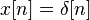$x[n] = delta[n]$

where δ[n] is the Kronecker delta impulse. The impulse response for an FIR filter follows asbegin{align} h[n] &= sum_{i=0}^{N}b_i delta[n-i] \ &= b_n. end{align}

The Z-transform of the impulse response yields the transfer function of the FIR filterbegin{align} H(z) &= Z{h[n]} \ &= sum_{n=-infty}^{infty} h[n] z^{-n} \ &= sum_{n=0}^{N}b_n,z^{-n}. end{align}

FIR filters are clearly BIBO stable, since the output is a sum of a finite number of finite multiples of the input values, so can be no greater than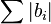$sum |b_i|$ times the largest value appearing in the input.

A FIR filter has a number of useful properties which sometimes make it preferable to an infinite impulse response filter. FIR filters:

• Are inherently stable. This is due to the fact that all the poles are located at the origin and thus are located within the unit circle.
• Require no feedback. This means that any rounding errors are not compounded by summed iterations. The same relative error occurs in each calculation.
• They can be designed to be linear phase, which means the phase change is proportional to the frequency. This is usually desired for phase-sensitive applications, for example crossover filters, and mastering, where transparent filtering is adequate.

A moving-average filter is a very simple FIR filter. The filter coefficients are found via the following equation:$b_{i}=frac{1}{N+1}$ for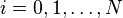$i=0,1,dots,N$

To provide a more specific example, we select the filter order:$N=2$

The impulse response of the resulting filter is: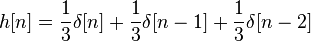$h[n]=frac{1}{3}delta[n]+frac{1}{3}delta[n-1]+frac{1}{3}delta[n-2]$

The following figure shows the block diagram of such a second-order moving-average filter.Block diagram of a simple FIR filter (3-tap filter in this case)

To discuss stability and spectral topics we take the z-transform of the impulse response:$H(z)=frac{1}{3}+frac{1}{3}z^{-1}+frac{1}{3}z^{-2}=frac{1}{3}frac{z^{2}+z+1}{z^{2}}$

The following figure shows the pole-zero diagram of the filter. Two poles are located at the origin, and two zeros are located at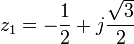$z_{1}=-frac{1}{2}+jfrac{sqrt{3}}{2}$,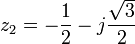$z_{2}=-frac{1}{2}-jfrac{sqrt{3}}{2}$The frequency response is:$H(e^{jomega})=frac{1}{3}+frac{1}{3}e^{-jomega}+frac{1}{3}e^{-j2omega}$

The following figure shows the absolute value of the frequency response. Clearly, the moving-average filter leaves low frequencies unaffected and blocks high frequencies. This is a typical low-pass filter characteristic.The following figure shows the phase response.Infinite impulse response

Infinite impulse response (IIR) is a property of signal processing systems. Systems with that property are known as IIR systems or when dealing with electronic filter systems as IIR filters. They have an impulse response function which is non-zero over an infinite length of time. This is in contrast to finite impulse response filters (FIR) which have fixed-duration impulse responses. The simplest analog IIR filter is an RC filter made up of a single resistor (R) feeding into a node shared with a single capacitor (C). This filter has an exponential impulse response characterized by an RC time constant.

IIR filters may be implemented as either analog or digital filters. In digital IIR filters, the output feedback is immediately apparent in the equations defining the output. Note that unlike with FIR filters, in designing IIR filters it is necessary to carefully consider “time zero” case in which the outputs of the filter have not yet been clearly defined.

Design of digital IIR filters is heavily dependent on that of their analog counterparts because there are plenty of resources, works and straightforward design methods concerning analog feedback filter design while there are hardly any for digital IIR filters. As a result, mostly, if a digital IIR filter is going to be implemented, first, an analog filter (e.g. Chebyshev filter, Butterworth filter, Elliptic filter) is designed and then it is converted to digital by applying discretization techniques such as Bilinear transform or Impulse invariance.

In practice, electrical engineers find IIR filters to be fast and cheap, but with poorer bandpass filtering and stability characteristics than FIR filters.

Example IIR filters include the Chebyshev filter, Butterworth filter, and the Bessel filter.

We start the discussion by stating the difference equation which defines how the input signal is related to the output signal:begin{align} y[n] & = b_{0} x[n] + b_{1} x[n-1] + cdots + b_{P} x[n-P] \ & - a_{1} y[n-1] - a_{2} y[n-2] - cdots - a_{Q} y[n-Q] end{align}

where:

•$P$ is the feedforward filter order
•$b_{i}$ are the feedforward filter coefficients
•$Q$ is the feedback filter order
•$a_{i}$ are the feedback filter coefficients
•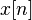$x[n]$ is the input signal
•$y[n]$ is the output signal.

A more condensed form of the difference equation is: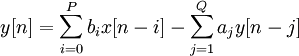$y[n] = sum_{i=0}^P b_{i}x[n-i] - sum_{j=1}^Q a_{j} y[n-j]$

which, when rearranged, becomes:$sum_{j=0}^Q a_{j} y[n-j] = sum_{i=0}^P b_{i}x[n-i]$

if we let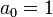$a_0 = 1$.

To find the transfer function of the filter, we first take the Z-transform of each side of the above equation, where we use the time-shift property to obtain: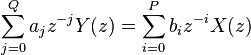$sum_{j=0}^Q a_{j} z^{-j} Y(z) = sum_{i=0}^P b_{i} z^{-i} X(z)$

We define the transfer function to be:begin{align} H(z) & = frac{Y(z)}{X(z)} \ & = frac{sum_{i=0}^P b_{i} z^{-i}}{sum_{j=0}^Q a_{j} z^{-j}} end{align}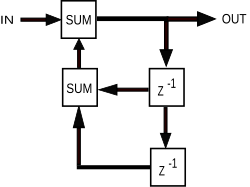Simple IIR filter block diagram

A typical block diagram of an IIR filter looks like the following. The z − 1 block is a unit delay. The coefficients and number of feedback/feedforward paths is implementation-dependent.

The transfer function allows us to judge whether or not a system is bounded-input, bounded-output (BIBO) stable. To be specific, the BIBO stability criteria requires all poles of the transfer function to have an absolute value smaller than one. In other words, all poles must be located within a unit circle in the z-plane.

The poles are defined as the values of z which make the denominator of H(z) equal to 0: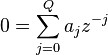$0 = sum_{j=0}^Q a_{j} z^{-j}$

Clearly, if$a_{j}ne 0$ then the poles are not located at the origin of the z-plane. This is in contrast to the FIR filter where all poles are located at the origin, and is therefore always stable.

IIR filters are sometimes preferred over FIR filters because an IIR filter can achieve a much sharper transition region roll-off than FIR filter of the same order.

Let the transfer function of a filter H be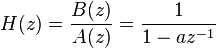$H(z) = frac{B(z)}{A(z)} = frac{1}{1 - a z^{-1}}$ with ROC a < | z | and 0 < a < 1

which has a pole at a, is stable and causal. The time-domain impulse response is

h(n) = anu(n)

which is non-zero for n > = 0.

SOURCE : WIKIPEDIA.org tentunya

Iklan

7 thoughts on “FIR & IIR”

1.fariddjunks berkata:

Melalui tulisan ini saya nyatakan tulisan ini sangat membosankan, merusak mata, meracuni pikiran, dan menghancurkan generasi muda.. huahaha… nice blog, tapi susah dibaca.. (kebanyakan warna item..)

2.panji berkata:

Bahas ttg FIR dan IIR nya in bahasa dunk..
hehe supaya bs lbh dimengerti.thx

3.rizasaputra berkata:

waduh.. iya deh mas panji, kapan-kapan klo sempet sy translate, rada males uy hehe

4.Odonicrippich berkata:

I agreed with you

5.kikirizki berkata:

Hahahaha…
Makasih2 nih, kakak
Dpt kuliah gratis, harus dimanfaatkan sebelum UTS PSD Sabtu depan 😀

6.mirza1302 berkata:

sangat membingungkan…

7.mirza1302 berkata:

sangat membingungkan….